Ex 5.1

Chapter 5 Class 12 Continuity and Differentiability
Serial order wise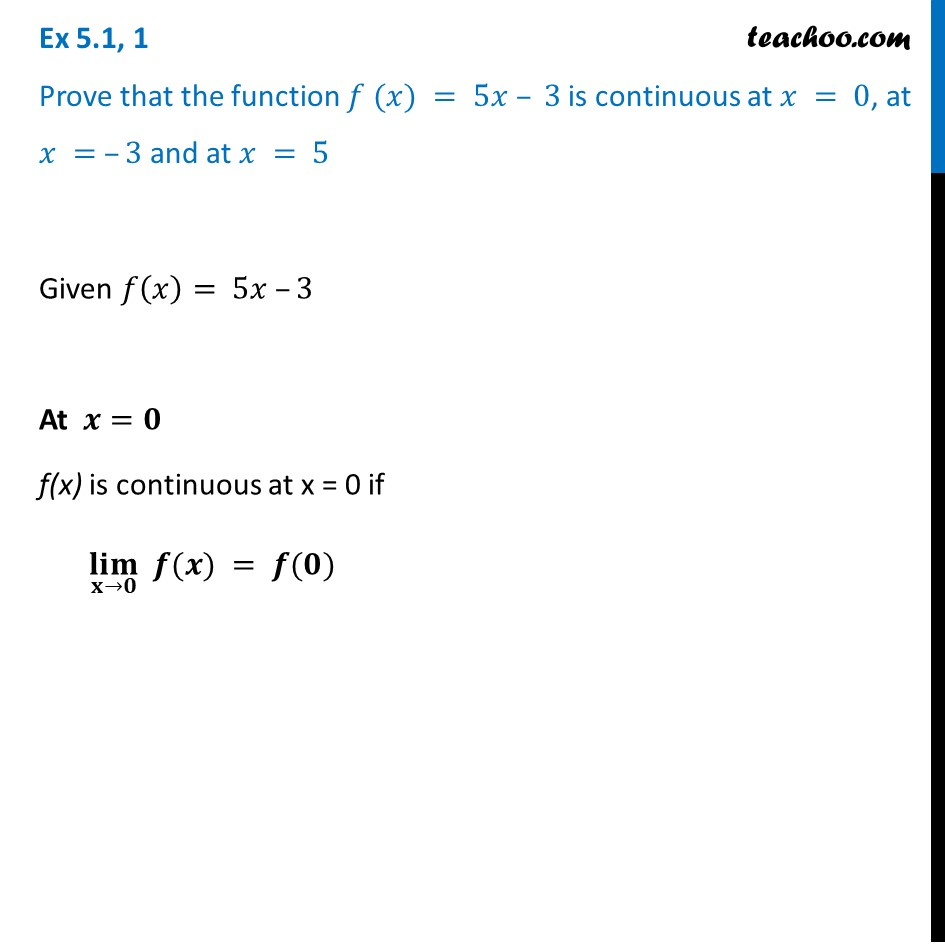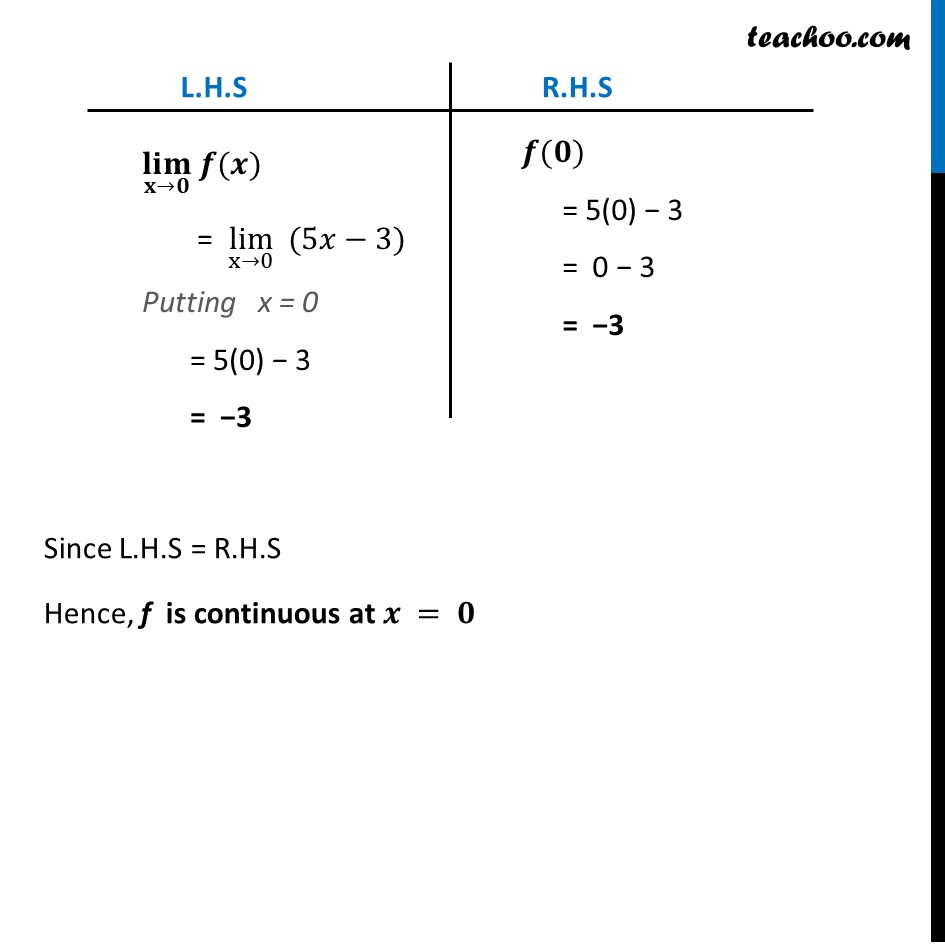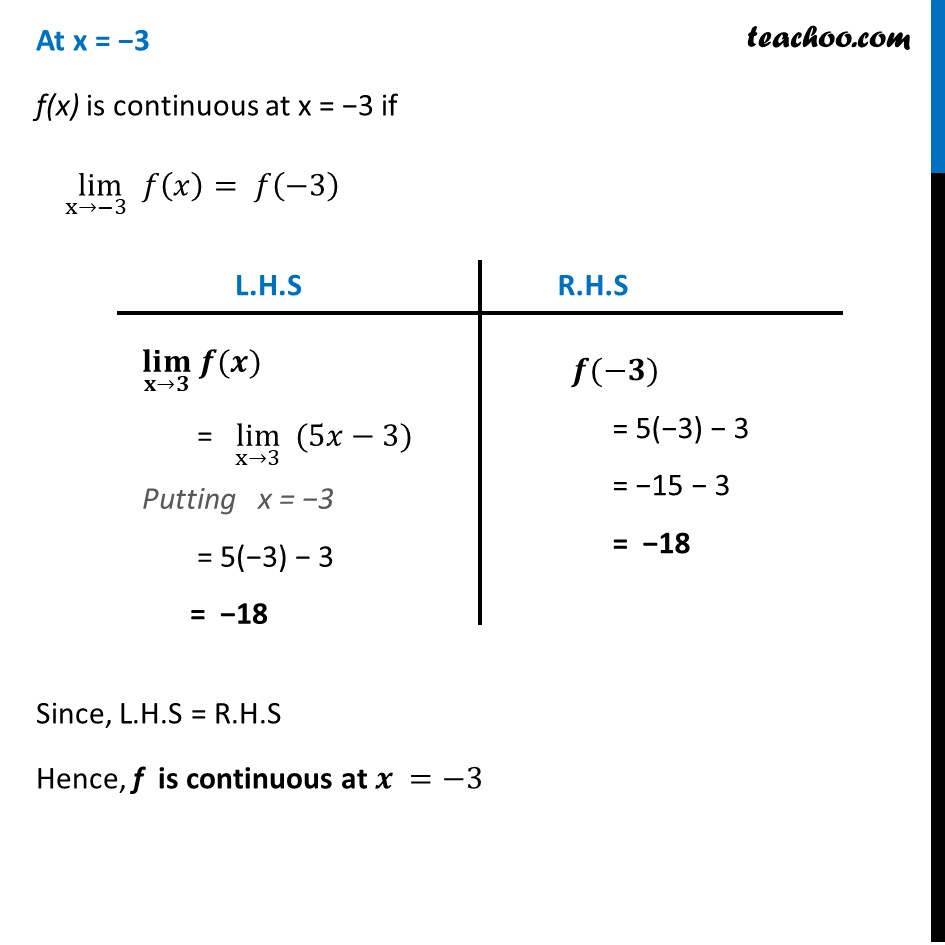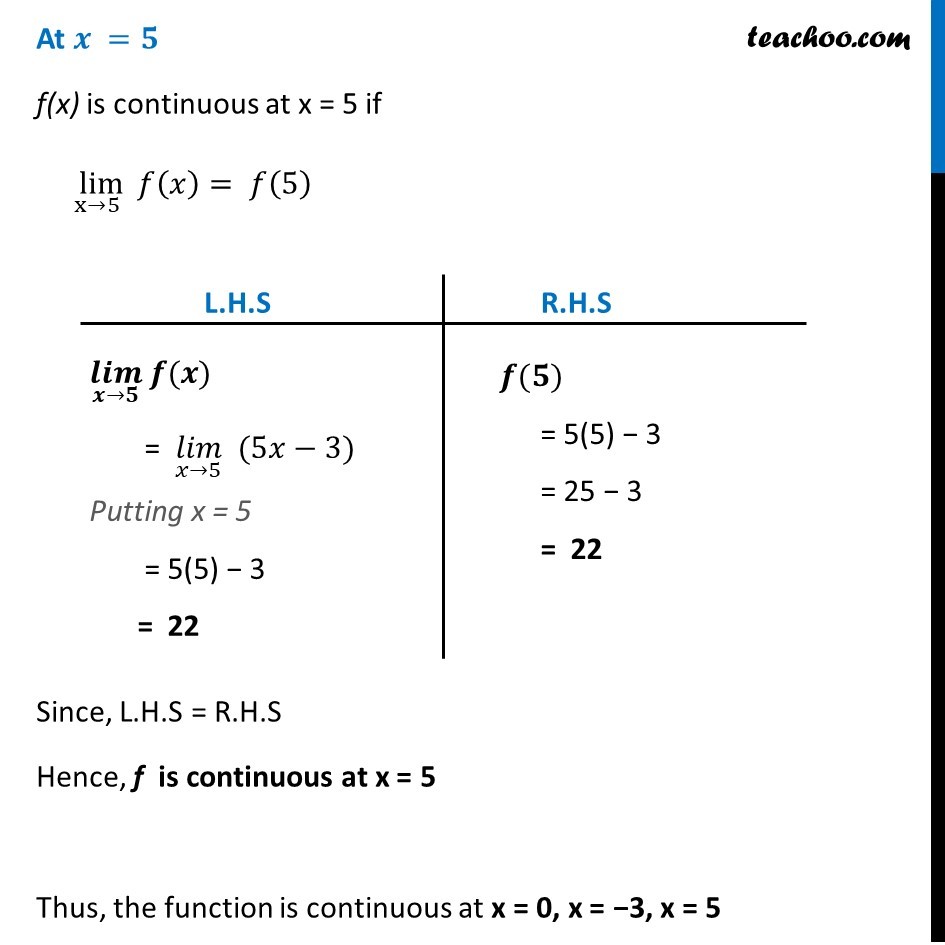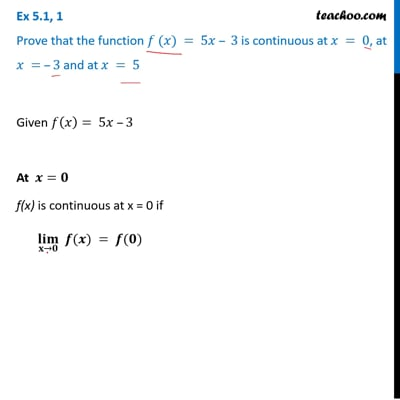This video is only available for Teachoo black users

Solve all your doubts with Teachoo Black (new monthly pack available now!)

### Transcript

Ex 5.1, 1 Prove that the function 𝑓 (𝑥) = 5𝑥 – 3 is continuous at 𝑥 = 0, at 𝑥 = –3 and at 𝑥 = 5 Given 𝑓(𝑥)= 5𝑥 –3 At 𝒙=𝟎 f(x) is continuous at x = 0 if (𝐥𝐢𝐦)┬(𝐱→𝟎) 𝒇(𝒙) = 𝒇(𝟎) (𝐥𝐢𝐦)┬(𝐱→𝟎) 𝒇(𝒙) "= " lim┬(x→0) " "(5𝑥−3) Putting x = 0 = 5(0) − 3 = −3 𝒇(𝟎) = 5(0) − 3 = 0 − 3 = −3 Since L.H.S = R.H.S Hence, f is continuous at 𝒙 = 𝟎 At x = −3 f(x) is continuous at x = −3 if ( lim)┬(x→−3) 𝑓(𝑥)= 𝑓(−3) Since, L.H.S = R.H.S Hence, f is continuous at 𝒙 =−3 (𝐥𝐢𝐦)┬(𝐱→𝟑) 𝒇(𝒙) "= " lim┬(x→3) " "(5𝑥−3) Putting x = −3 = 5(−3) − 3 = −18 𝒇(−𝟑) = 5(−3) − 3 = −15 − 3 = −18 At 𝒙 =𝟓 f(x) is continuous at x = 5 if ( lim)┬(x→5) 𝑓(𝑥)= 𝑓(5) Since, L.H.S = R.H.S Hence, f is continuous at x = 5 Thus, the function is continuous at x = 0, x = −3, x = 5 (𝒍𝒊𝒎)┬(𝒙→𝟓) 𝒇(𝒙) "= " (𝑙𝑖𝑚)┬(𝑥→5) " "(5𝑥−3) Putting x = 5 = 5(5) − 3 = 22 𝒇(𝟓) = 5(5) − 3 = 25 − 3 = 22